# RBSE Solutions For Class 12 Physics Chapter 11: Ray Optics | Textbook Important Questions & Answers

The answers are provided for all the questions of Chapter 11 Physics of RBSE Class 12. Students can go through these questions to understand the concepts better and score well in the board examination and entrance examinations for various professional courses. These solutions are provided by the team of experts and offer the best and accurate solutions to the questions. Do check BYJU’S RBSE Class 12 solutions page to get the answers for textbook questions of all subjects.

## Multiple Choice Questions

Q1: In spherical mirrors, we consider only paraxial rays for forming the images, because

1. They are easy to work for a geometric purpose
2. They consist of the most intense incident light
3. They form point image of the point object
4. They produce a minimum dispersion

Q2: An object is placed at 30 cm distance from a concave mirror of focal length 20 cm, then nature, magnification of the image will be.

1. Real, -2
2. Apparent, -2
3. Real, +2
4. Apparent, +2

Q3: The refractive index for infrared rays

1. Is equal to ultraviolet rays
2. Is equal to red colour rays
3. Less than ultraviolet rays
4. Greater than ultraviolet rays

Q4: Total internal reflection occurs, when

1. Light travels from optically rarer medium to optically denser medium
2. Light travels from an optically denser medium to optically rarer medium
3. Refractive index of both the medium are equal
4. Refractive index of both the medium are different

Q5: When an object is placed at a distance of 20 cm from the concave lens, then a small image is formed. Then the correct statement will be

1. The image will be inverted
2. Image may be real
3. Image is formed at 20 cm distance
4. The focal length of the lens may be less than 20 cm

## Very Short Questions

Q1: What is the focal length of the plane mirror?

Answer: The focal length of the plane mirror is infinite.

Q2: Which lens has a magnification less than 1?

Answer: Concave mirror has a magnification less than 1 since the image formed concave mirror is always erect and smaller than the object.

Q3: What is the cause of refraction?

Answer: Refraction is caused because the speed of light is different in a different medium.

Q4: What is the cause of mirage seen in the desert in summer?

Answer: The reason for mirage formation is total internal reflection.

Q5: For an equal angle of incidence, refraction angle for these media A, B and C are 150, 250 and 350 respectively. In which medium speed of light is minimum?

Refractive index of the medium

μ = sin i/sinr

Here sin i = constant

Therefore μ ∝ 1/sinr

Since

rA=150

rB = 250

rC = 350

Sin rA く SinrBく SinrC

Therefore μA ＞μB ＞μC

So the velocity of light is minimum in medium A

Q6: Write the working principle of an optical fibre.

Answer: Optical fibre works on the principle of total internal refraction.

Q7: What is the relation between i and e when the prism is in the position of minimum deviation?

Answer: In the condition of minimum deviation

Angle of incidence(i) = Angle of emergence(e)

Q8: Two lenses convex and concave of equal focal length are coaxially in contact. What will be the resultant focal length?

1/F = 1/f1 + 1/f2 = 1/f – 1/f =0

Therefore f = 1/0 = ∞

Or f = ∞ (infinite)

Q9: Why does the Sun appear reddish at sunrise and sunset?

Answer: The Sun appears reddish at sunrise and sunset due to scattering.

Q10: What is the cause of the rainbow?

Answer: Rainbow is caused by a phenomenon called dispersion. The sunlight is dispersed by the water drops present in the atmosphere after rain.

Q11: What is myopia? Which lens is used for its correction?

Answer: Myopia is a defect caused in the eye in which the person can see nearby objects clearly and objects far cannot be seen clearly. This can be corrected using a concave lens.

Q12: On what factor, the intensity of scattered light depends?

Answer: Scattering depends on the wavelength of light

Q13: What type of lens is used in a simple microscope?

Answer: In a simple microscope a convex lens of less focal length is used.

Q14: How can you differentiate between a compound microscope and a telescope just by looking at it?

Answer: In a microscope, the aperture of the objective lens is smaller than the eyepiece, while in a telescope, it is vice versa.

Q1: An object AB is placed in front of a concave mirror as shown in the figure.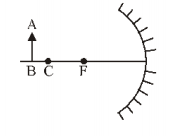(i) Draw a ray diagram to form an image

(ii) If half of the apparatus of the mirror is blackened, how is the position and intensity of the image affected?

(i) the ray diagram for the image is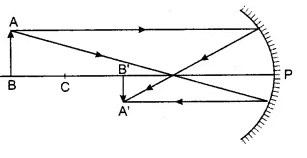(ii) If half of the apparatus of the mirror is blackened then the position of the image formed will not change but the intensity of the image will be reduced to half.

Q2: Write the uses of spherical mirrors.

1. A concave mirror is used as a shaving mirror because it forms an erect and magnified image of the object when it is kept closer to the object.
2. Doctors use concave mirrors as a head mirror. These mirrors reflect the light into the mouth and ear.
3. Concave mirrors are used as reflectors in a solar cooker, torches and table lamps.
4. Convex mirrors are used as rearview mirrors in vehicles.

Q3: Establish the relation between focal length and radius of curvature for a spherical mirror.

Let us consider the convex mirror. Let the focal length of the convex mirror is f and its radius of curvature is R. OA is an incident ray parallel to the principal axis and AS is the reflected ray which appears to come from the focus F. The normal to the principal axis is given by AN.

According to the law of reflection angle of incidence is the angle to reflection.

i = r = θ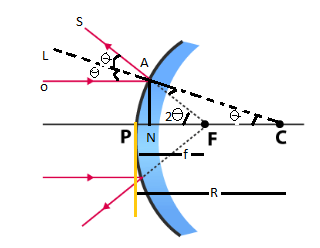The angles ㄥFAC = ㄥLAS = θ (because they are vertically opposite angles)

The angles ㄥACF = ㄥOAL = θ ( they are corresponding angles)

Exterior angleㄥ AFP = interior angle (ㄥFAC + ㄥACF)

Therefore ㄥ AFP = θ + θ =2θ

For the right angle triangle ΔANC

tan θ = AN/NC

If θ is very small, then tan θ = θ and the point N is very close to P. Therefore NC≈ PC ≈ R

So we have θ = AN/NC = AN/R————————(1)

Similarly for the triangle ΔANF

tan 2θ = AN/FN

If 2θ is very small, then tan 2θ = 2θ and the point N is very close to P. Therefore NF≈ PF ≈ f

2θ = AN/FP = AN/f —————————————-(2)

From equation 1 and 2 we have

2θ = 2AN/R = AN/f

2/R = 1/f

f = R/2

Therefore, Focal length = Radius of curvature/2

Q4:(i) Why does the sun appear reddish at sunrise and sunset?

(ii) For which colour, is the refractive index of the medium minimum and maximum?

(i) According to Rayleigh’s scattering law, the intensity of scattered light is proportional to the fourth root of the wavelength.

I ∝ 1/λ4

From the law, we know that smaller is the wavelength of light, more will be the scattering.

At sunrise and sunset, the sun is near the horizon and the light rays have to travel a larger distance than at noon when the sun is overhead. The blue region of light with lower wavelength is completely scattered away by the molecules of air. The higher wavelength red region is least scattered and reaches our eyes.

(ii) The refractive index of the prism is maximum for violet colour and minimum for the red colour

Q5: (i) What is the relation between critical angle and refractive index of a medium?

(ii) Do the critical angle depend on the colour of light? Explain.

(i) The relation between critical angle (c) and refractive index (μ) is given bya and b are the two medium through which light ray travels.

(ii) The refractive index depends on the colour of light so critical angle also depends on colour of light.

Q6: On what factors does the focal length of the lens depend?

The focal length of the lens depends on the radius of curvature, the refractive index of lens material and the refractive index of the material in which the lens is placed.

Q7: How can we increase the magnifying power of a compound microscope?

Answer: The focal length of the object and the eyepiece of the compound microscope should be small.

Q8: What do you understand by scattering of light? Write down its uses in daily life?

When the light rays are incident on the particles with a wavelength much smaller than the wavelength of light then the light gets absorbed by the particle and later gets emitted in all possible directionss. Thus, scattering is a process by which light is deviated from its path due to its interaction with the particles of the medium through which it passes.

Uses of scattering of light in daily life

1. It is used to determine the molecular weight of the substance
2. Air pollution analysis
3. In traffic signal

Q9: Define power of a lens and write down its unit. For two coaxially contracted lenses, derive the relation

1/f = 1/f1 +1/f2

Power of a lens: It is the deviation produced by the lens to the rays coming parallel to the principal axis at a unit distance from the principal axis. The SI unit of power of lens is Dioptre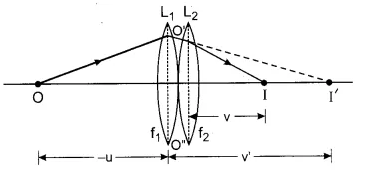Let us take two lenses L1 and L2 of focal length f1 and f2 respectively. A point object O is at a distance u in front and I is the image. The image I’ formed by the first lens acts as the virtual image for the second lens.

From the lens makers formula we have

For first lens

1/v’ – 1/u = 1/f1—————————–(1)

For second lens

1/v – 1/v’ = 1/f2—————————–(2)

Adding both the equation we have

(1/v’) – (1/u )+ (1/v) – (1/v’) =(1/f1) +(1/f2)————(3)

Let us consider the focal length of combination of lenses to be F,

Therefore 1/F = (1/v) – (1/u )—————————(4)

On comparing equation (3) and (4) we have

1/F = (1/f1) +(1/f2)

## Essay Type Questions

Q1: Define a spherical mirror. Find the relation between object distance, image distance and focal length for it.

Spherical Mirror: A spherical mirror is like a portion of a hollow sphere cut and polished on one side. The spherical mirror reflects light.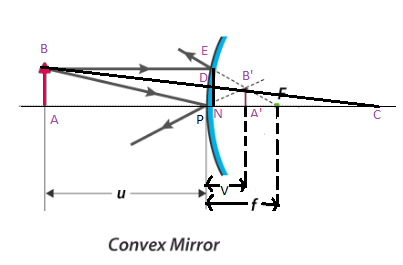ΔABC and ΔA’B’C are identical. In these triangles

AB/A’B’ = AC/A’C———————(1)

Similarly for the triangle, ΔENF and ΔA’B’F are also similar triangles. Hence in these triangles

EN/A’B’ = NF/A’F ——————(2)

Therefore EN = AB

AB/A’B’ = NF/A’F ——————(3)

If the mirror aperture is small then the point N is very close to P.

Therefore NF≈ PF

Hence AB/A’B’ = PF/A’F———(4)

Now comparing equation (1) and (4) we have

AC/A’C = PF/A’F ——————(5)

(AP + PC)/(PC – PA’) = PF/(PF-PA’)——–(6)

According to the sign convention we have

AP = -u

PA’ = +v

PF = +f

PC = +R = 2f

Substituting these values in equation (6) we get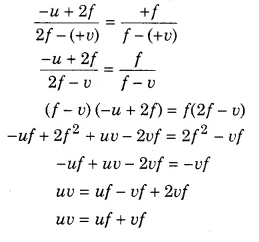Dividing the above equation by uvf we get

uv/uvf = uf/uvf + vf/uvf

I/f =(1/v) + (1/u)

Q2: What types of lenses are there? Establish the relation between object distance, image distance and focal length of a lens.

The classification of a lens depends on how the light rays bend when they pass through the lens. The two main types of lenses are:

• Convex Lens (Converging)
• Concave Lens (Diverging)

Convex lenses are thick in the middle and thinner at the edges. A concave lens is flat in the middle and thicker at the edges. A convex lens is also known as the converging lens as the light rays bend inwards and converge at a point, which is known as focal length. On the other hand, the concave lens is also known as a diverging lens because it bends the parallel light rays outward and diverges them at the focal point.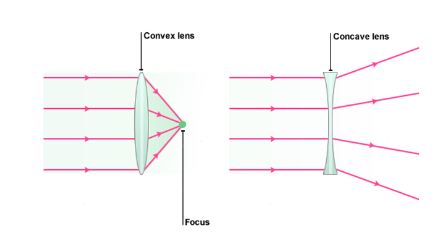Simple lens and compound are the two classifications of lenses. Simple lenses are different from compound lenses based on their surface of curvature. Following are the different types of simple lenses.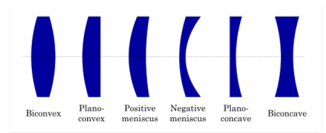Compound lenses are those constructed out of a combination of different simple lenses. The lens types used to build a compound lens may have different refractive indices and other properties. The placement of lenses are such that they lie on a single axis, and properties like focal length are calculated again for the new compound lens.

Relation between object distance, image distance and focal length of a lens

Consider a convex lens with an optical centre O. Let F be the principle focus and f be the focal length. An object AB is held perpendicular to the principal axis at a distance beyond the focal length of the lens. A real, inverted magnified image A’B’ is formed as shown in the figure.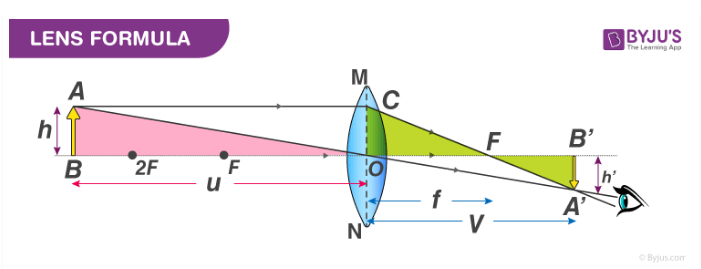From the above figure, we notice that ΔABO and ΔA’B’O are similar

Therefore,

A’B/AB = OB’/OB ———————————–(1)

Similarly, ΔA’B’F and ΔOCF are similar, hence

A’B’/OC = FB’/OF

But, OC =AB

Therefore A’B’/AB = FB’/OF———————-(2)

Equating (1) and (2) we get

OB’/OB = FB’/OF =( OB’ – OF)/OF

Substituting the sign convention, we get

OB = -u, OB’ =v, OF = f

(v/-u) = (v-f)/f

vf = -uv + uf or uv = uf – vf

Dividing both the sides by uvf, we get

uv/uvf = (uf/uvf) – (vf/uvf)

1/f = (1/v) – (1/u)

The above equation is known as the Lens formula